# Bicylindrical coordinates

(diff) ← Older revision | Latest revision (diff) | Newer revision → (diff)

The numbers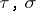andrelated to the rectangular Cartesian coordinates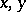andby the formulas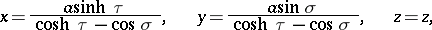where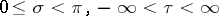. The coordinate surfaces are: the family of pairs of circular cylinders with parallel axes (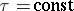), the family of circular cylinders orthogonal to the former (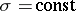), and the planes (). The system of bicylindrical coordinates is obtained as the result of translation of the system of bipolar coordinates in the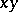-plane parallel to the-axis.

The Lamé coefficients (cf. Lamé coefficients) are:The Laplace operator is: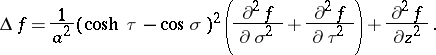How to Cite This Entry:
Bicylindrical coordinates. Encyclopedia of Mathematics. URL: http://encyclopediaofmath.org/index.php?title=Bicylindrical_coordinates&oldid=16217
This article was adapted from an original article by D.D. Sokolov (originator), which appeared in Encyclopedia of Mathematics - ISBN 1402006098. See original article